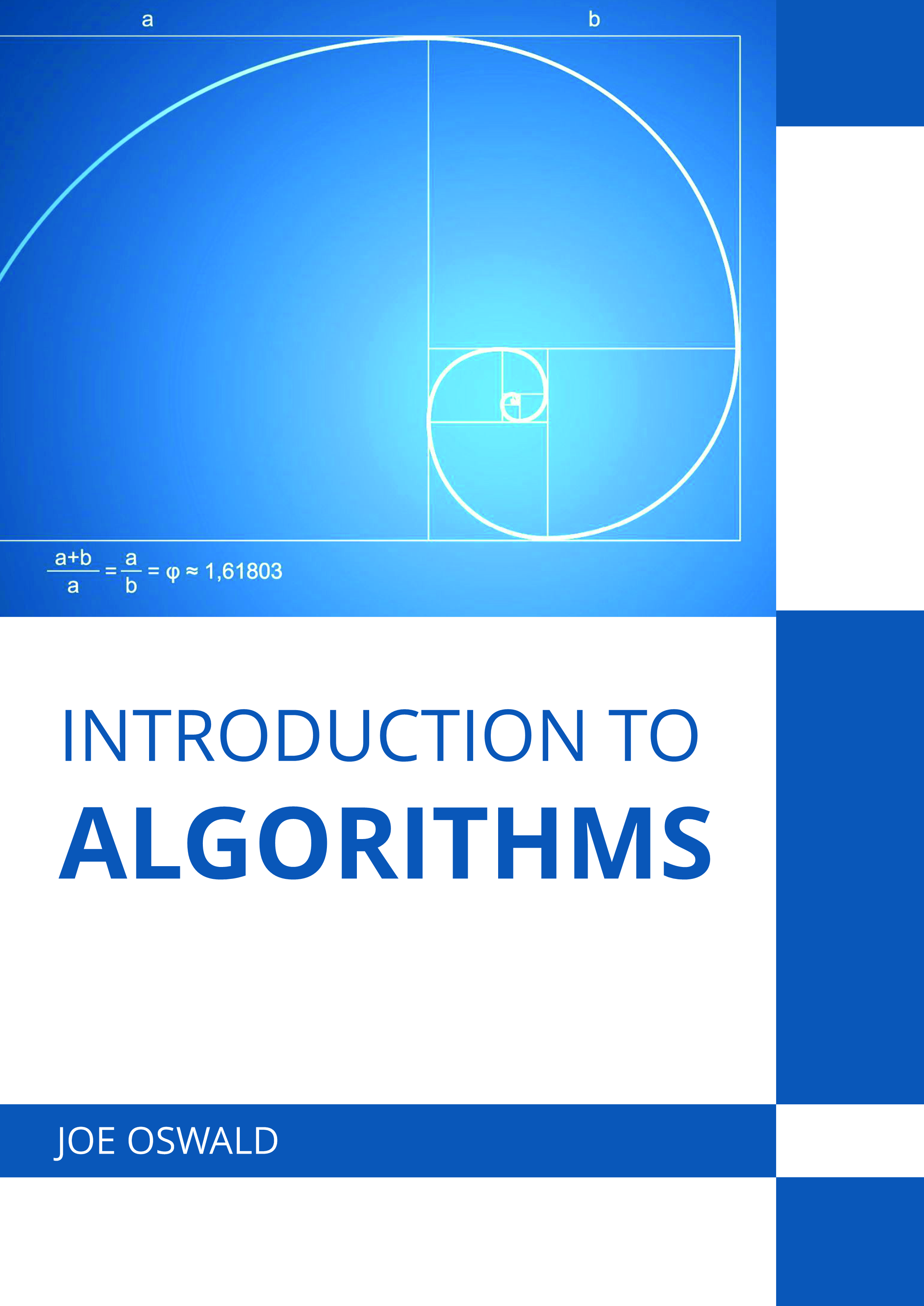# Books /Introduction to Algorithms

BROWSE BY

### Introduction to Algorithms9781639893003

Author

Joe Oswald

English

##### Publication Year

(2022)

Category :

Computer Science & Information Technology - Computer Science

\$ 156

##### Description

Finite sequences of well-defined instructions are known as algorithms. These are used to perform various computations, calculations, data processing and solve classes of problems. Algorithms are unambiguous and computer implementable in nature. These can be represented in many different forms such as flow charts, natural languages, drakon-charts, pseudo code and control tables. These representations can be classified into high level description, implementation description and formal description. Algorithms can be broadly categorized on the basis of implementation, design and complexity. The means of implementation of algorithms are further divided into recursive algorithms, logical algorithms, serial algorithms, parallel algorithms, deterministic algorithms, exact and quantum algorithm. According to the level of complexity, they can be divided into constant time, logarithmic time, linear time, polynomial time and exponential time algorithms. This book presents the complex subject of algorithms in the most comprehensible and easy to understand language. Different approaches, evaluations and methodologies and advanced studies on algorithms have been included herein. This book is a complete source of knowledge on the present status of this important field.